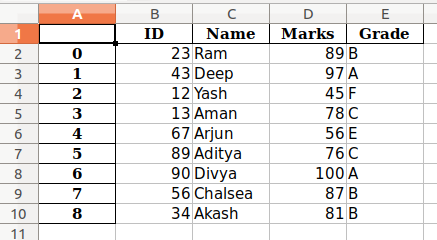# DataFrame.to_excel() method in Pandas

• Last Updated : 16 Jul, 2020

The to_excel() method is used to export the DataFrame to the excel file.  To write a single object to the excel file, we have to specify the target file name. If we want to write to multiple sheets, we need to create an ExcelWriter object with target filename and also need to specify the sheet in the file in which we have to write. The multiple sheets can also be written by specifying the unique sheet_name. It is necessary to save the changes for all the data written to the file.

Syntax:

Attention geek! Strengthen your foundations with the Python Programming Foundation Course and learn the basics.

To begin with, your interview preparations Enhance your Data Structures concepts with the Python DS Course. And to begin with your Machine Learning Journey, join the Machine Learning - Basic Level Course

```data.to_excel( excel_writer, sheet_name='Sheet1', \*\*kwargs )
```

Parameters:

• One can provide the excel file name or the Excelwrite object.
• By default the sheet number is 1, one can change it by input the value of argument “sheet_name”.
• One can provide the name of the columns to store the data by input the value of the argument “columns”.
• By default the index is labeled with numbers as 0,1,2 … and so on, one can change it by passing a sequence of the list for the value of the argument “index”.

Below is the implementation of the above method :

## Python3

 `# importing packages ``import` `pandas as pd `` ` `# dictionary of data ``dct ``=` `{``'ID'``: {``0``: ``23``, ``1``: ``43``, ``2``: ``12``, ``              ``3``: ``13``, ``4``: ``67``, ``5``: ``89``, ``              ``6``: ``90``, ``7``: ``56``, ``8``: ``34``}, ``      ``'Name'``: {``0``: ``'Ram'``, ``1``: ``'Deep'``, ``               ``2``: ``'Yash'``, ``3``: ``'Aman'``, ``               ``4``: ``'Arjun'``, ``5``: ``'Aditya'``, ``               ``6``: ``'Divya'``, ``7``: ``'Chalsea'``, ``               ``8``: ``'Akash'` `}, ``      ``'Marks'``: {``0``: ``89``, ``1``: ``97``, ``2``: ``45``, ``3``: ``78``, ``                ``4``: ``56``, ``5``: ``76``, ``6``: ``100``, ``7``: ``87``, ``                ``8``: ``81``}, ``      ``'Grade'``: {``0``: ``'B'``, ``1``: ``'A'``, ``2``: ``'F'``, ``3``: ``'C'``, ``                ``4``: ``'E'``, ``5``: ``'C'``, ``6``: ``'A'``, ``7``: ``'B'``, ``                ``8``: ``'B'``} ``    ``} `` ` `# forming dataframe``data ``=` `pd.DataFrame(dct) `` ` `# storing into the excel file``data.to_excel(``"output.xlsx"``)`

Output :In the above example,

• By default index is labeled as 0,1,…. and so on.
• As our DataFrame has columns names so columns are labeled.
• By default, it is saved in “Sheet1”.

My Personal Notes arrow_drop_up